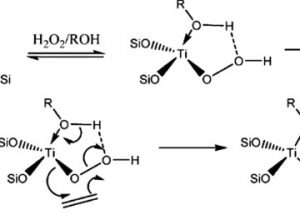# Modelling of transient kinetics in trickle bed reactors: Ethylene oxide production via hydrogen peroxide

Matias Alveara, Michele Emanuele Fortunato, Vincenzo Russo, Tapio Salmi, Martino Di Serio

Chemical Engineering Science, Volume 248, Part A, 2022, 117156, https://doi.org/10.1016/j.ces.2021.117156.

Abstract

Stationary kinetic methods are not sufficient for studying chemical processes in which solid heterogeneous catalysts and several phases (gas-liquid-solid) are involved, therefore transient experimental techniques are applied to reveal the molecular processes on the catalyst surfaces. Dynamic methods were used to study the kinetics of a heterogeneously catalyzed reaction i.e. the epoxidation of ethylene with hydrogen peroxide as the oxidizing agent and titanium silicalite (TS-1) as the heterogeneous catalyst. No detailed reaction mechanism and kinetic model has ever been proposed for this process. For the kinetic investigation, step response experiments were carried out in a laboratory-scale trickle bed reactor (TBR).

A dynamic isothermal model for the TBR was presented together with a dynamic kinetic model based on a proposed surface reaction mechanism, where the key step is the reaction between adsorbed hydrogen peroxide and dissolved ethylene from the liquid phase. The TBR model consisted of dynamic mass balance equations for the components present in the gas and liquid bulk phases as well as adsorbed species on the catalyst surface. The model formed a coupled system of parabolic partial differential equations (gas and liquid phases) and ordinary differential equations (catalyst surface phase). The coupled system of differential equations, describing the concentration profiles from the step response experiments were solved numerically by the method of lines by discretizing the reactor length coordinate with finite differences and solving the large system of ordinary differential equations by a backward difference algorithm suitable for stiff ordinary differential equations. The parameters for surface kinetics, mass transfer and fluid dynamics were determined by non-linear regression analysis using the maximum likelihood approach. The model was able to predict the transient and stationary behavior of the system.